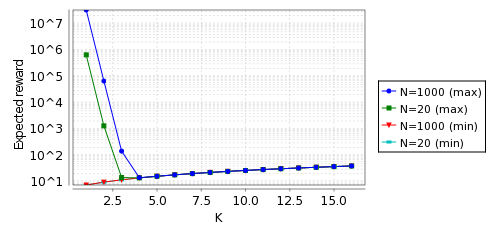# IPv4 Zeroconf Protocol

### Contents

Related publications: [KNPS06]

### Introduction

The widespread use of the Internet and its technologies has lead to the adoption of the Internet Protocol (IP) suite not only in a global context, but also for local, ad-hoc networks, such as domestic networks which can connect devices ranging from microwave ovens, televisions, DVD players and hand-held devices. In such a local context, it is desirable that the user is not required to manage the initialisation of the network, which would involve configuring static IP addresses for each device or setting up a DHCP server for the dynamic assignment of IP addresses. This desirable property requires that each device must be able to configure its own unique, local IP address (that is, it must be self-configuring), and that the network must be able to maintain the unique assignment of IP addresses to each device even in the situation in which appliances can be connected to or disconnected from the network.

These concerns have lead to the development of a dynamic configuration protocol for IPv4 addresses [CAG02], which offers a distributed "plug-and-play" solution in which address configuration is managed by individual devices when they are connected to the network. Upon being connected to the network, a device is first required to choose randomly an IP address from a pool of 65024 available addresses (the Internet Assigned Number Authority has allocated the addresses from 169.254.1.0 to 169.254.254.255 for the purpose of such link-local networks). Then the device sends messages to the other devices connected to the network, asking whether any of those devices are currently using the chosen IP address. If no reply is received even after the devices resend such messages three more times, then the device starts using the IP address, initially sending two more messages asserting that the device is claiming the address. The draft standard also specifies the manner in which the device should respond to incoming messages from other devices; the messages will either require the device to reconfigure a new IP address, or, in certain circumstances, the device can defend its ownership of the IP address. We note that the reason for the repeated transmission of messages is primarily to ameliorate the effect of message loss. The protocol is both probabilistic and timed: probability is used most prominently in the randomised selection process of an IP address by a device, but also features in the probability of message loss, whereas timing issues primarily arise in the definition of the time periods that elapse between repeated retransmissions of the same message.

### The Protocol

A device, henceforth called a host, that wishes to configure an IP address first selects randomly an address out of a given set of 65024 possible addresses. The host then sends four ARP (address resolution protocol) packets, called probes, to all of the other hosts of the network. Probes contain the IP address selected by the host, operate as requests to use the address, and are sent at two second intervals. A host which is already using the address will respond with an ARP reply packet, asserting its claim to the address, and the original host will restart the protocol by reconfiguring its chosen address and sending new probes. If the host sends four probes without receiving an ARP reply packet, then it commences to use the chosen IP address. It sends confirmations of this fact to the other hosts of the network in the form of more messages called gratuitous ARPs. Two gratuitous ARPs are sent in total, also at two second intervals, after the IP address has started to be used. The protocol has an inherent degree of redundancy, for example with regard to the number of repeated ARP packets sent, in order to cope with message loss. Indeed, message loss makes possible the undesirable situation in which two or more hosts use the same IP address simultaneously.

A host which has commenced to use an IP address must reply to ARP packets containing the same IP addresses that it receives from other hosts. In the case that a host using an IP address received a probe with the same address, then it sends an ARP reply packet, and continues using the address. Instead, if the host receives a gratuitous ARP containing the IP address that it is using currently, then it will either defend its IP address, or defer to the host which sent the conflicting ARP packet. The host may only defend its address if it has not received a previous conflicting ARP packet within the previous ten seconds; otherwise it is forced to defer. A defending host replies by the sending a gratuitous ARP, thereby indicating that it is using the IP address. A deferring host does not send a reply; instead, it ceases using its current IP address, reconfigures another IP address and restarts the protocol.

As indicated by the draft standard [CAG02], the protocol can operate in the context of any IEEE 802 network technology, or similar technology in which the transfer rate is at least 1Mb/s, which has a round-trip latency of at most one second, and which supports ARP, such as Ethernet.

### The Model

As in [ZV03], we assume a "broadcast"-based communication medium with no routers (for example, a single wire), in which messages arrive in the order in which they are sent. In contrast to the analysis of the protocol included in Bohnenkamp et al. [BSHV03], we model the possibility that a device could surrender an IP address that it is using to another host; and in contrast to timed-automata-based analysis of Zhang and Vaandrager [ZV03], we model some important probabilistic characteristics of the protocol, and consider parameters more faithful to the standard (such as the maximum number of times a device can witness an ARP packet with the same IP address as that which is wishes to use before "backing off" and remaining idle for at least 1 minute).

When modelling the dynamic configuration protocol, we use a probabilistic timed automaton model which refers to integer variables and urgent events. Integer variables with bounded ranges, which can be tested within enabling conditions and reset by edge distributions, can be represented syntactically within the probabilistic timed automaton framework above by encoding the values of such variables within locations [Tri98]; instead, urgent events require minor adjustments to the semantics of probabilistic timed automata (see [KNS02a]).

Using the digital clocks approach presented in [KNPS06], we obtain a PRISM model (a finite-state Markov decision process) which preserves both probabilistic and expected reachability properties of the dense time semantic model of the PTA.

##### Modelling Assumptions

The hosts: we consider two scenarios: in the first the number, N, of (abstract) hosts which have chosen their IP addresses equals 1000, and in the second N equals 20. In each case we suppose that these abstract hosts always defend their addresses (do not reconfigure) and one concrete host trying to configure an IP address. Therefore, when the concrete host picks a new address, the probability of this address being fresh (not in use by another host) is (8128-N)/8128. We also assume that the concrete host never picks the same IP address twice as this happens with a very small probability. Also, the (continuous) uniform choice over [0,2] (made before the concrete host sends its first probe) is abstracted to a discrete uniform choice over {0,1,2}.

IP addresses: following from the above assumptions we require only three abstract IP addresses (0,1 and 2) which correspond to the following sets of IP addresses:

 0 - the IP addresses of the abstract hosts which the concrete host previously tried to configure; 1 - an IP address of an abstract host which the concrete host is currently trying to configure; 2 - a fresh IP address which the concrete host is currently trying to configure.

Message: following the standard we suppose that it takes between 0 and 1 second to send a message between any hosts (where the choice is nondeterministic). Since the abstract hosts have already picked there IP address and always defend them, we can suppose that the concrete host will never receive probes. It then follows that we do not need to record they type of message being sent only the IP address in the message and whether it is from the concrete host to the abstract hosts or vice versa.

We suppose that the hosts have buffers to store the messages that they want to send. The concrete host can send a message to all the abstract hosts at the same time, while one message can be sent either to or from the concrete host at a time. We have chosen the size of the buffers such that the probability of any other the buffers becoming full is negligible.

In the standard [CAG02] there is no mention of what the host should do with the messages, which corresponding to choosing its current IP address (i.e. the probes and two gratuitous ARP packets specified in the standard) which are in its out buffer (i.e. those that have yet to be sent), when it decides to choose a new IP address. However, when the host does reconfigure, unless it picks the same IP address which happens with a very small probability, these messages are not relevant and, in fact, can be harmful by both slow down the network and making hosts reconfigure when they do not need to. We therefore considered two different versions of the network: one where the host does not do anything about these messages (No_Reset) and the another where the host deletes these messages when it it about to choose a new IP address (Reset).

##### Probabilistic Timed Automata Representing the Concrete Host

The model for the concrete host is shown below.The host commences in the location RECONF (the double border indicates that the initial location). The location RECONF is a committed location, and therefore must be left immediately. In RECONF, the host chooses a new IP address by moving to the location CHOOSE if it has experienced less than ten address collisions, and to CHOOSEWAIT otherwise. These transitions are labelled with the event reset to inform the environment that the host's buffer is to be reset (all messages in its buffer are to be removed).

In both CHOOSE and CHOOSEWAIT, the address selection is represented by the assignment iph := RAND(1,2), which corresponds to the host randomly selecting an IP address. That is, with probability N/65024 the host sets iph equal to 1 (selects an IP address already in use) and, with probability (65024-N)/65024, sets iph equal to 2 (selects a fresh IP address). Note that, in CHOOSEWAIT, since the host has already experienced at least ten address collisions, it waits 60 seconds before choosing a new address. The assignment to the clock x (a uniform choice between 0,1,2), which labels the transitions from CHOOSE and CHOOSEWAIT to WAITSP, approximates the random delay of between 0 and 2 made by the host before sending the first probe.

In the location WAITSP, the host sends K probes at 2 second intervals (denoted by the self-loop labelled with send). The host may also receive packets by means of the event rec. If it receives a packet which has a different IP address (ipiph), then the host ignores the packet (and remains in WAITSP); however, if the packet has the same address, the host immediately reconfigures (moves to RECONF).

After sending the Kth probe, the host remains in location WAITSP for 2 seconds before moving to WAITSG. The host then sends two ARPs separated by a delay of 2 seconds. Note that the variable probes is used to count these ARPs. After these ARPs have been sent, the host moves to USE. However, if while in WAITSG the host receives a packet with the same IP address, it moves to RESPOND. In this location, the host can decide to reconfigure (return to RECONF), or defend its IP address (by sending an ARP packet) if it has either not yet defended the address (defend=0) or if 10 seconds have passed since it previously defended the address (y10). This defence takes the form of the sending of a defending packet, as denoted by the send labelled transition from RESPOND to WAITSG.

##### Probabilistic Timed Automata Representing the Environment

The model for the environment is shown below.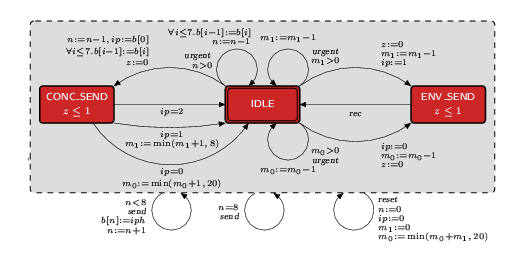The dotted box labelled with three transitions which surrounds the model denotes that these transitions are available in all of the locations of the model. More precisely, in all locations, the environment may: receive a send event from the concrete host and, if the host's buffer is not full (n < 8), add the corresponding packet to the buffer (otherwise it is lost); receive a reset event and clear the buffer of the concrete host (n:=0) and, since we assume that the concrete host will never choose the same IP address twice, set the IP address in any packet being sent or to be sent to type 0 (i.e. ip:=0, m1:=0 and m0:=min(m0{+}m1,20)).

The behaviour of the environment commences in the location IDLE. The transition which probabilistically moves to either IDLE or CONC_SEND corresponds to the sending of a packet from the concrete host's buffer. The urgent labelling denotes that the transition should be taken as soon as it is enabled, i.e. it should be taken as soon as there is a packet to send. Similarly, the transitions which move probabilistically to either IDLE or ENV_SEND correspond to an abstract host sending a packet, and are again urgent. There are two such transitions, since the address in the packet can either be of type 0 (m0>0) or 1 (m1>0). For each of these transitions, the loop (remaining in IDLE) corresponds to the packet being lost by the medium, while the other edge corresponds to the packet being sent correctly (therefore the required buffers are updated when one of these transitions is taken). Note that, since each of these transitions corresponds to a message from a different host, when more than one of these transitions is enabled, there is a nondeterministic choice as to which one is taken. We vary the probability of message loss depending on the verification instance. Once in either CONC_SEND or ENV_SEND, after a delay of between 0 and 1 seconds, the model returns to IDLE; this corresponds to the message taking between 0 and 1 seconds to send.

##### PRISM Models

The PRISM model for is given below (this also includes details on how to construct the No_Reset model).

```// IPv4: PTA model with digitial clocks
// one concrete host attempting to choose an ip address
// when a number of (abstract) hosts have already got ip addresses
// gxn/dxp/jzs 02/05/03

// reset or noreset model
const bool reset;

//-------------------------------------------------------------

// we suppose that
//   and always defend their addresses
// - the concrete host never picks the same ip address twice
//   (this can happen only with a verys small probability)

// under these assumptions we do not need message types because:
// 1) since messages to the concrete host will never be a probe,
//    this host will react to all messages in the same way
// 2) since the abstract hosts always defend their addresses,
//    all messages from the host will get an arp reply if the ip matches

// following from the above assumptions we require only three abstract IP addresses
// (0,1 and 2) which correspond to the following sets of IP addresses:

// 0 - the IP addresses of the abstract hosts which the concrete host
//     previously tried to configure
// 1 - an IP address of an abstract host which the concrete host is
//     currently trying to configure
// 2 - a fresh IP address which the concrete host is currently trying to configure

// if the host picks an address that is being used it may end up picking another ip address
// in which case there may still be messages corresponding to the old ip address
// to be sent both from and to the host which the host should now disregard
// (since it will never pick the same ip address)

// to deal with this situation: when a host picks a new ip address we reconfigure the
// messages that are still be be sent or are being sent by changing the ip address to 0
// (an old ip address of the host)

// all the messages from the abstract hosts for the 'old' address (in fact the
// set of old addresses since it may have started again more than once)
// can arrive in any order since they are equivalent to the host - it ignores then all

// also the messages for the old and new address will come from different hosts
// (the ones with that ip address) which we model by allowing them to arrive in any order
// i.e. not neccessarily in the order they where sent

//-------------------------------------------------------------
// model is an mdp
mdp

//-------------------------------------------------------------
// VARIABLES
const int N; // number of abstract hosts
const int K; // number of probes to send
const double loss; // probability of message loss

// PROBABILITIES
const double old = N/65024; // probability pick an ip address being used
const double new = (1-old); // probability pick a new ip address

// TIMING CONSTANTS
const int CONSEC = 2;  // time interval between sending consecutive probles
const int TRANSTIME = 1; // upper bound on transmission time delay
const int LONGWAIT = 60; // minimum time delay after a high number of address collisions
const int DEFEND = 10;

const int TIME_MAX_X = 60; // max value of clock x
const int TIME_MAX_Y = 10; // max value of clock y
const int TIME_MAX_Z = 1;  // max value of clock z

// OTHER CONSTANTS
const int MAXCOLL = 10;  // maximum number of collisions before long wait
// size of buffers for other hosts
const int B0 = 20;  // buffer size for one abstract host
const int B1 = 8;  // buffer sizes for all abstract hosts

//-------------------------------------------------------------
// ENVIRONMENT - models: medium, output buffer of concrete host and all other hosts
module environment

// buffer of concrete host
b_ip7 : [0..2]; // ip address of message in buffer position 8
b_ip6 : [0..2]; // ip address of message in buffer position 7
b_ip5 : [0..2]; // ip address of message in buffer position 6
b_ip4 : [0..2]; // ip address of message in buffer position 5
b_ip3 : [0..2]; // ip address of message in buffer position 4
b_ip2 : [0..2]; // ip address of message in buffer position 3
b_ip1 : [0..2]; // ip address of message in buffer position 2
b_ip0 : [0..2]; // ip address of message in buffer position 1
n : [0..8]; // number of places in the buffer used (from host)

// messages to be sent from abstract hosts to concrete host
n0  : [0..B0]; // number of messages which do not have the host's current ip address
n1  : [0..B1]; // number of messages which have the host's current ip address

b : [0..2]; // local state
// 0 - idle
// 1 - sending message from concrete host
// 2 - sending message from abstract host

z : [0..1]; // clock of environment (needed for the time to send a message)

ip_mess : [0..2]; // ip in the current message being sent
// 0 - different from concrete host
// 1 - same as the concrete host and in use
// 2 - same as the concrete host and not in use

// suppose that the host cannot choose the same ip address
// (since happens with very small probability).
// Therefore all messages will have a different ip address,
// i.e. all n1 messages become n0 ones.
// Note this include any message currently being sent (ip is set to zero 0)
[reset] true -> (n1'=0) & (n0'=min(B0,n0+n1)) // abstract buffers
& (ip_mess'=0) // message being set
& (n'=(reset)?0:n) // concrete buffer
& (b_ip7'=0)
& (b_ip6'=0)
& (b_ip5'=0)
& (b_ip4'=0)
& (b_ip3'=0)
& (b_ip2'=0)
& (b_ip1'=0)
& (b_ip0'=0);
// note: prevent anything else from happening when reconfiguration needs to take place

// time passage (only if no messages to send or sending a message)
[time] l>0 & b=0 & n=0 & n0=0 & n1=0 -> (b'=b); // cannot send a message
[time] l>0 & b>0 & z<1 -> (z'=min(z+1,TIME_MAX_Z)); // sending a message

// get messages to be sent (so message has same ip address as host)
[send] l>0 & n=0 -> (b_ip0'=ip) & (n'=n+1);
[send] l>0 & n=1 -> (b_ip1'=ip) & (n'=n+1);
[send] l>0 & n=2 -> (b_ip2'=ip) & (n'=n+1);
[send] l>0 & n=3 -> (b_ip3'=ip) & (n'=n+1);
[send] l>0 & n=4 -> (b_ip4'=ip) & (n'=n+1);
[send] l>0 & n=5 -> (b_ip5'=ip) & (n'=n+1);
[send] l>0 & n=6 -> (b_ip6'=ip) & (n'=n+1);
[send] l>0 & n=7 -> (b_ip7'=ip) & (n'=n+1);
[send] l>0 & n=8 -> (n'=n); // buffer full so lose message

// start sending message from host
[] l>0 & b=0 & n>0 -> (1-loss) : (b'=1) & (ip_mess'=b_ip0)
& (n'=n-1)
& (b_ip7'=0)
& (b_ip6'=b_ip7)
& (b_ip5'=b_ip6)
& (b_ip4'=b_ip5)
& (b_ip3'=b_ip4)
& (b_ip2'=b_ip3)
& (b_ip1'=b_ip2)
& (b_ip0'=b_ip1) // send message
+ loss : (n'=n-1)
& (b_ip7'=0)
& (b_ip6'=b_ip7)
& (b_ip5'=b_ip6)
& (b_ip4'=b_ip5)
& (b_ip3'=b_ip4)
& (b_ip2'=b_ip3)
& (b_ip1'=b_ip2)
& (b_ip0'=b_ip1); // lose message

// start sending message to host
[] l>0 & b=0 & n0>0 // different ip
-> (1-loss) : (b'=2) & (ip_mess'=0) & (n0'=n0-1) + loss : (n0'=n0-1);
[] l>0 & b=0 & n1>0 // same ip
-> (1-loss) : (b'=2) & (ip_mess'=1) & (n1'=n1-1) + loss : (n1'=n1-1);

// finish sending message from host
[] l>0 & b=1 & ip_mess=0 -> (b'=0) & (z'=0) & (n0'=min(n0+1,B0)) & (ip_mess'=0);
[] l>0 & b=1 & ip_mess=1 -> (b'=0) & (z'=0) & (n1'=min(n1+1,B1)) & (ip_mess'=0);
[] l>0 & b=1 & ip_mess=2 -> (b'=0) & (z'=0) & (ip_mess'=0);

// finish sending message to host
[rec] l>0 & b=2 -> (b'=0) & (z'=0) & (ip_mess'=0);

endmodule

//-------------------------------------------------------------
// CONCRETE HOST
module host0

x : [0..TIME_MAX_X]; // first clock of the host
y : [0..TIME_MAX_Y]; // second clock of the host

coll : [0..MAXCOLL]; // number of address collisions
probes : [0..K]; // counter (number of probes sent)
mess : [0..1]; // need to send a message or not
defend : [0..1]; // defend (if =1, try to defend IP address)

ip : [1..2]; // ip address (1 - in use & 2 - fresh)

l : [0..4] init 1; // location
// 0 : RECONFIGURE
// 1 : RANDOM
// 2 : WAITSP
// 3 : WAITSG
// 4 : USE

// RECONFIGURE
[reset] l=0 -> (l'=1);

[rec] (l=1) -> true; // get message (ignore since have no ip address)
// small number of collisions (choose straight away)
[] l=1 & coll<MAXCOLL -> 1/3*old : (l'=2) & (ip'=1) & (x'=0)
+ 1/3*old : (l'=2) & (ip'=1) & (x'=1)
+ 1/3*old : (l'=2) & (ip'=1) & (x'=2)
+ 1/3*new : (l'=2) & (ip'=2) & (x'=0)
+ 1/3*new : (l'=2) & (ip'=2) & (x'=1)
+ 1/3*new : (l'=2) & (ip'=2) & (x'=2);
// large number of collisions: (wait for LONGWAIT)
[time] l=1 & coll=MAXCOLL & x<LONGWAIT -> (x'=min(x+1,TIME_MAX_X));
[]     l=1 & coll=MAXCOLL & x=LONGWAIT -> 1/3*old : (l'=2) & (ip'=1) & (x'=0)
+ 1/3*old : (l'=2) & (ip'=1) & (x'=1)
+ 1/3*old : (l'=2) & (ip'=1) & (x'=2)
+ 1/3*new : (l'=2) & (ip'=2) & (x'=0)
+ 1/3*new : (l'=2) & (ip'=2) & (x'=1)
+ 1/3*new : (l'=2) & (ip'=2) & (x'=2);

// WAITSP
// let time pass
[time]  l=2 & x<2 -> (x'=min(x+1,2));
// send probe
[send] l=2 & x=2  & probes<K -> (x'=0) & (probes'=probes+1);
// sent K probes and waited 2 seconds
[] l=2 & x=2 & probes=K -> (l'=3) & (probes'=0) & (coll'=0) & (x'=0);
// get message and ip does not match: ignore
[rec] l=2 & ip_mess!=ip -> (l'=l);
// get a message with matching ip: reconfigure
[rec] l=2 & ip_mess=ip
-> (l'=0) & (coll'=min(coll+1,MAXCOLL)) & (x'=0) & (probes'=0);

// WAITSG (sends two gratuitious arp probes)
// time passage
[time] l=3 & mess=0 & defend=0 & x<CONSEC
-> (x'=min(x+1,TIME_MAX_X));
[time] l=3 & mess=0 & defend=1 & x<CONSEC
-> (x'=min(x+1,TIME_MAX_X)) & (y'=min(y+1,DEFEND));

// receive message and same ip: defend
[rec] l=3 & mess=0 & ip_mess=ip & (defend=0 | y>=DEFEND)
-> (defend'=1) & (mess'=1) & (y'=0);
// receive message and same ip: defer
[rec] l=3 & mess=0 & ip_mess=ip & (defend=0 | y<DEFEND)
-> (l'=0) & (probes'=0) & (defend'=0) & (x'=0) & (y'=0);
// receive message and different ip
[rec] l=3 & mess=0 & ip_mess!=ip -> (l'=l);

// send probe reply or message for defence
[send] l=3 & mess=1 -> (mess'=0);
// send first gratuitous arp message
[send] l=3 & mess=0 & x=CONSEC & probes<1 -> (x'=0) & (probes'=probes+1);
// send second gratuitous arp message (move to use)
[send] l=3 & mess=0 & x=CONSEC & probes=1
-> (l'=4) & (x'=0) & (y'=0) & (probes'=0);

// USE (only interested in reaching this state so just loop)
[] l=4 -> true;

endmodule

//-------------------------------------------------------------

// reward structure
const double err; // cost associated with using a IP address already in use

rewards
[time] true : 1;
[send] l=3 & mess=0 & y=CONSEC & probes=1 & ip=1 : err;
endrewards

```

Note that, to change between the Reset and No_Reset models, change the number of probes sent (K), size of the buffers, the number of abstract hosts or the timing delays of the protocol one need only change the constants given at start of the files.

The table below shows statistics for the MTBDD which represents each model we have built, in terms of the number of states, the total number of nodes and the number of leaves (terminal nodes), for both the models as the number of probes varies. Note that, the probability of message loss and the number of abstract hosts (assuming each is greater than 0) does not influence these statistics.

 K No_Reset Reset States Nodes Leaves States Nodes Leaves 1 31,954 73,318 6 451 679 6 2 89,586 207,825 6 670 997 6 3 179,774 416,688 6 879 1,305 6 4 307,768 712,132 6 1088 1,613 6

The table below gives the times taken to construct the models. There are two stages. Firstly, the system description (in our module based language) is parsed and converted to the MTBDD representing it. Secondly, reachability is performed to identify non-reachable states and the MTBDD is filtered accordingly. Reachability is performed using a BDD based fixpoint algorithm. The number of iterations for this process is also given.

 K No_Reset Reset Time (s) Iter.s Time (s) Iter.s 1 6.703 530 1.133 138 2 10.42 317 1.171 142 3 14.52 288 1.226 146 4 35.09 334 1.205 150

### Model Checking

We now report on the model checking results obtained using PRISM when considering a number of different parameters. We consider three different performance measures:

In each case, we calculate upper and lower bounds on these measures which since the PRISM model is an abstraction will give upper and lower bounds on an actual implementation. In the case of the expected cost, as in [BSHV03], this is the expected time to start using an IP address with an additional cost (E) associated with using an IP address which is already in use (we consider two different values for E, namely 1e+6 and 1e+12).

### Probability of the host using an IP address already in use

To calculate the minimum and maximum probability of the host using an IP address already in use by checking the following properties:

Pmin=?[ true U (l=4 & ip=1) ] and Pmax=?[ true U (l=4 & ip=1) ]

### Probability of not choosing a fresh address by time T

To calculate the minimum and maximum probability of not choosing a fresh address by time T we add the following module to our PRISM source code (where BT):

```const int B; // time bound
// timer
module timer

t : [0..B+1];

[time] t<=B -> t'=min(t+1,B+1);
[done] l=4 -> t'=T+1;

endmodule
```

and then check the following properties:

Pmin=?[ !(l=4 & ip=2) U t>T ] and Pmax=?[ !(l=4 & ip=2) U t>T ]

In the graphs below, we haver plotted the minimum and maximum probability of not choosing a fresh IP address by time T, as T varies from 0 to 50 seconds for the model Reset when N=1,000.

• Probability of message loss equals 0.1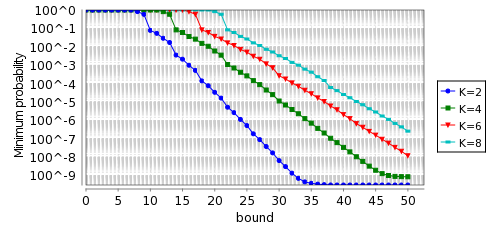• Probability of message loss equals 0.001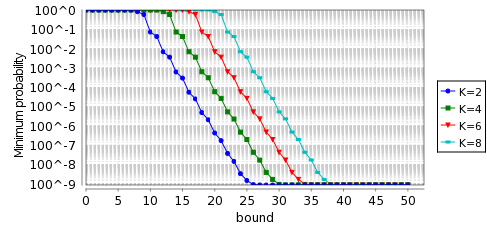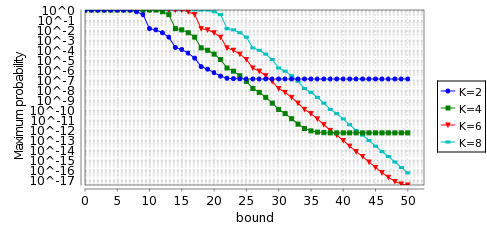### Expected cost of choosing an IP address

To calculate the expected costs we check the following properties:

Rmin=?[ F l=4 ] and Rmax=?[ F l=4 ]

In the graphs below, when the probability of message loss equals 0.1 and 0.001, we give the minimum and maximum cost of choosing an IP address for the model Reset.

• Probability of message loss equals 0.1 and E=1e+6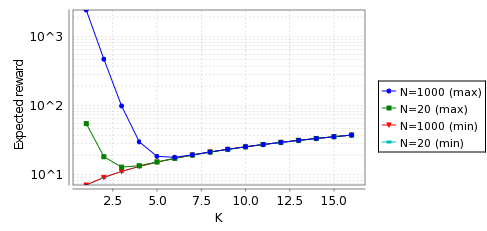• Probability of message loss equals 0.1 and E=1e+12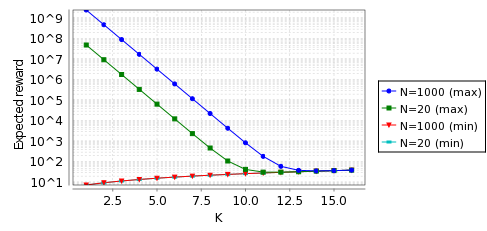• Probability of message loss equals 0.001 and E=1e+6• Probability of message loss equals 0.001 and E=1e+12